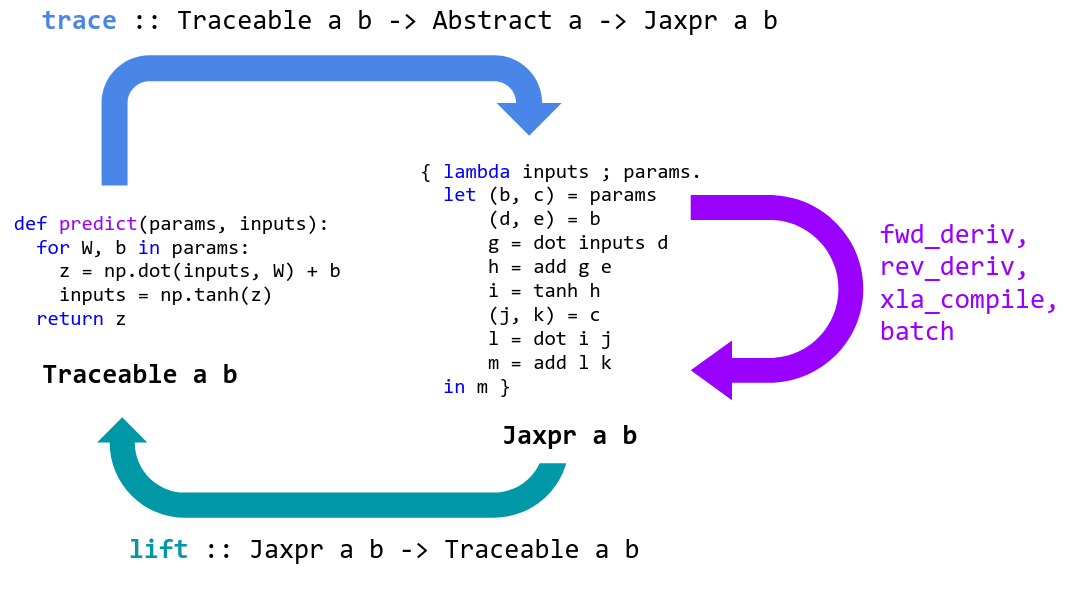#DAY 5
0
AI & Data

## Day 5 Jax 來拯救：numpy-based 的動態計算圖

Autograd 就像是 PyTorch 的 autograd module，但在這裡沒有另外生成 Tensor 物件。Autograd 所需要的就是由使用者提供具有計算邏輯的函式，接著使用模組階級的 `grad` 函式來生成另一個計算梯度的函式。`grad`內部運作的方式則是：

1. 先 trace 使用者函式的計算過程，在 tracing 的過程，將原函式拆成數個 primitive 小運算元。
2. 接著當使用者函式被呼叫時，第一步驟建立的 Tracer 會將函式表現式抽象化（abstraction），如對於函式的輸入，用符號代表而非真正傳入的數值。這個部分將會產出使用者函示的 syntax tree。
3. autograd 模組內會維持一個表格，其 key 值是運算元，而 value 值是該運算元對應計算梯度的函式。透過增加一層 wrapper 在使用者傳遞的函式上，將會記錄過程中使用的所有計算元，並完成計算圖。``````import jax.numpy as np # Thinly-wrapped version of Numpy

def sigmoid(x):
return 0.5 * (np.tanh(x / 2.) + 1) # sigmoid function for binary classification

def logistic_predictions(weights, inputs):
# Outputs probability of a label being true according to logistic model.
return sigmoid(np.dot(inputs, weights))

def training_loss(weights, targets):
# Training loss is the negative log-likelihood of the training labels.
preds = logistic_predictions(weights, inputs)
# cross-entropy for binary cases
label_probabilities = preds * targets + (1 - preds) * (1 - targets)
return -np.sum(np.log(label_probabilities))

# Build a toy dataset.
inputs = np.array([[0.52, 1.12,  0.77], # x
[0.88, -1.08, 0.15],
[0.52, 0.06, -1.30],
[0.74, -2.49, 1.39]])
targets = np.array([True, True, False, True]) #y
# Define a function that returns gradients of training loss using Autograd.

# Optimize weights using gradient descent.
weights = np.array([0.0, 0.0, 0.0]) # giving initial values
print("Initial loss:", training_loss(weights, targets))
for i in range(100):
print("Trained loss:", training_loss(weights, targets))

#Initial loss: 2.7725887
#Trained loss: 1.0672708
``````

`TypeError: training_loss() missing 1 required positional argument: 'targets'`

# Jax 是 Autograd on steroids

XLA 是 google 自家的 just-in-time (jit) 編譯器，主要針對線性代數的部分進行加速。透過 jit 編譯器來編譯 python 原始碼已經不是新鮮事，Numba 就是使用 llvm 來完成 python 的 byte code。然而 python 的原始碼透過 XLA 編譯成機械碼後，可以配置到 GPU 甚至到 TPU 上面。下圖就是取自官方網站，透過 XLA 編譯後的原始碼，會加快 1 到 1.15 倍的速度。``````grad_fun = jit(grad(logprob_fun))  # compiled gradient evaluation function